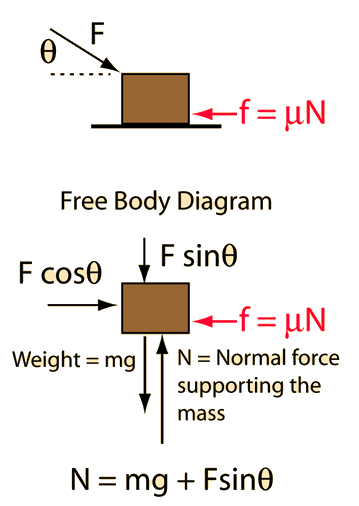# Mass with force at angleApplication of Newton's second law to a single mass.
An applied force F=N at an angle of degrees is opposed by friction with friction coefficient mu =. If it acts on mass m=kg then the normal force is N and the frictional resistance force is N. The horizontal component of the applied force is N and the net force is = N. Newton's second law becomes:

### Net force = mass x acceleration

Net force = N = kg x m/s²

You may edit any of the data. When finished with data entry, click on the quantity you desire to calculate in the formula above. Quantities will not be forced to be consistent until you click on the desired quantity.

In U.S. common units this becomes:
Net force = lb = slug x ft/s²

In this system the weight on the earth's surface islbs.

### Remove friction

Index

Newton's laws

Standard mechanics problems

 HyperPhysics***** Mechanics R Nave
Go Back